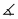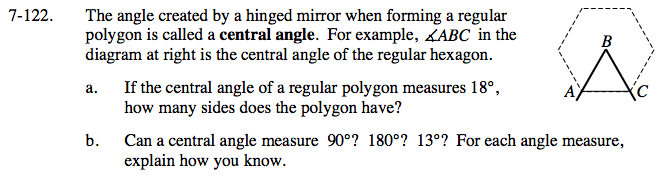### Home > GC > Chapter 7 > Lesson 7.3.3 > Problem7-122

7-122.
1. The angle created by a hinged mirror when forming a regular polygon is called a central angle. For example,ABC in the diagram at right is the central angle of the regular hexagon. Homework Help ✎

1. If the central angle of a regular polygon measures 18°, how many sides does the polygon have?

2. Can a central angle measure 90°? 180°? 13°? For each angle measure, explain how you know.$\text{number of sides }=\; \frac{360}{18}$

Try drawing shapes that could have the central angle measurements that have been listed.